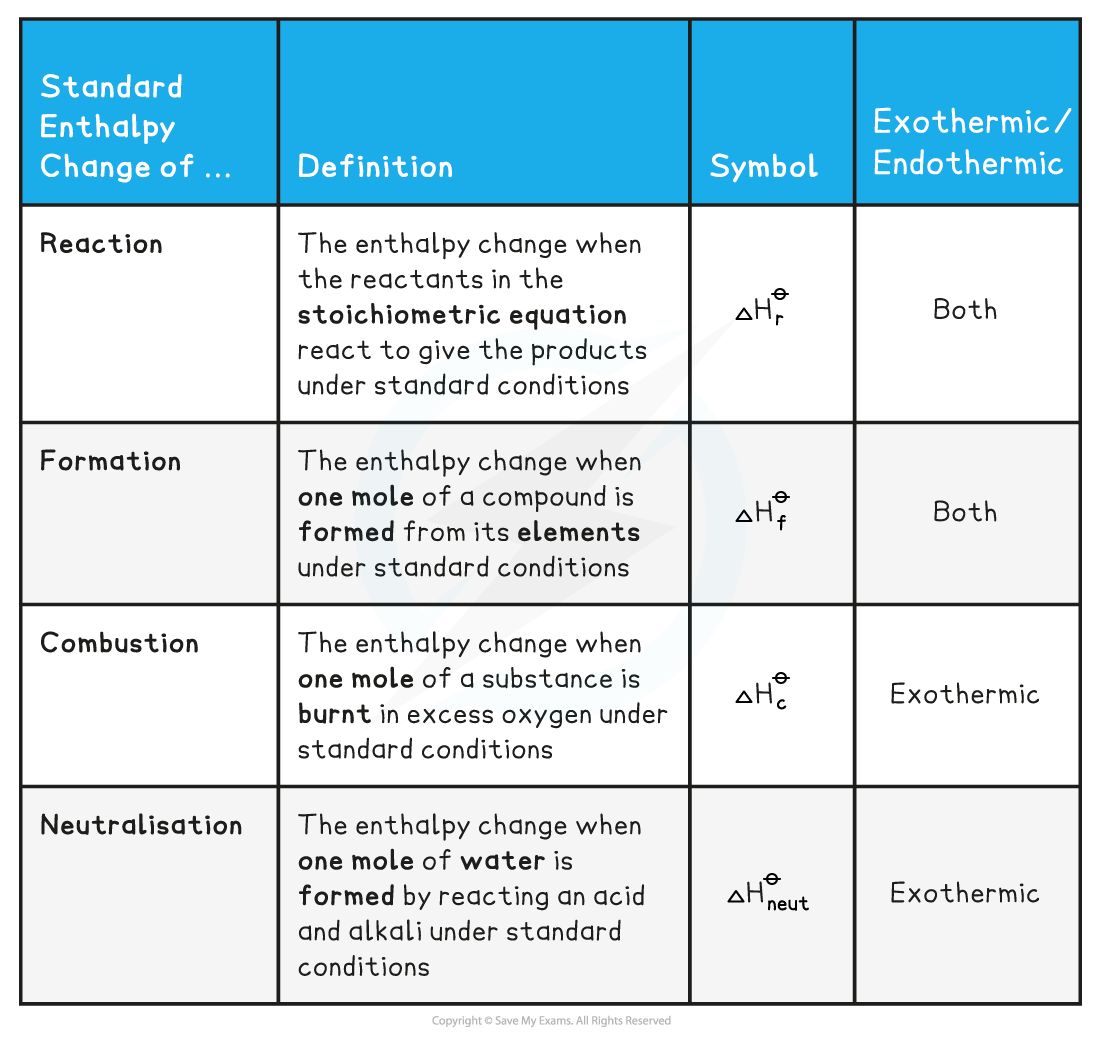# Edexcel A Level Chemistry:复习笔记1.8.1 Enthalpy Changes

### Enthalpy Change Definitions

• To be able to compare the changes in enthalpy between reactions, all thermodynamic measurements are carried out under standard conditions
• These standard conditions are:
• A pressure of 100 kPa (you may see some older exam questions that use a figure of 101 kPa; the exact figure is 101 325 Pa, but it has been simplified in the current syllabus for problem-solving purposes)
• A temperature of 298 K (25 oC)
• Each substance involved in the reaction is in its standard physical state (solid, liquid or gas)

• To show that a reaction has been carried out under standard conditions, the symbol Ꝋ is used
• ΔHꝊ = the standard enthalpy change

• There are a number of key definitions relating to enthalpy changes that you need to know

Enthalpy Definitions Table#### Exam Tip

You will see various enthalpy change symbols used with subtle changes, e.g.

ΔHcꝊ or ΔcHꝊ for enthalpy of combustion

Whichever symbol you use must have the following basic points:

• Δ to represent change
• H to represent enthlalpy
• Ꝋ to respresent standard conditions
• A symbol to represent the type of enthalpy change occurring, e.g.
• c for combustion
• f for formation
• neut for neutralisation
• r for reaction

#### Worked Example

Calculating the enthalpy change of reaction

One mole water is formed from hydrogen and oxygen, releasing 286 kJ of energy

H2 (g) + ½O2 (g) → H2O (I)      ΔHrꝊ = -286 kJ mol-1

Calculate ΔHrꝊ for the reaction below:

2H2 (g) + O2 (g) → 2H2O (I)

• Since two moles of water molecules are formed in the question above, the energy released is simply:
• ΔHrꝊ = 2 mol x (-286 kJ mol-1) = -572 kJ mol-1

#### Worked Example

Calculating the enthalpy change

Calculate ΔHfꝋ for the reaction below, given that ΔHfꝋ [Fe2O3(s)] = -824.2 kJ mol-1

4Fe(s) + 3 O2(g) → 2 Fe2O3(s)

• Since two moles of Fe2O3 (s) are formed the total change in enthalpy for the reaction above is:
• ΔHfꝊ =  2 x ( -824.2 kJ mol-1) = - 1648 kJ

#### Worked Example

Calculating enthalpy changes

Identify each of the following as ΔHrꝊ, ΔHfꝊ, ΔHcꝊ or ΔHneutꝊ

1. MgCO3 (s) → MgO (s) + CO2 (g)
2. C (graphite) + O2 (g) → CO2 (g)
3. HCl (aq) + NaOH (aq) → NaCl (aq) + H2O (I)

Answer 2: ΔHfꝊ as one mole of CO2 is formed from its elements in standard state and ΔHcꝊ as one mole of carbon is burnt in oxygen

Answer 3: ΔHneutꝊ as one mole of water is formed from the reaction between an acid and an alkali

#### Exam Tip

The ΔHfꝊ of an element in its standard state is zero.

For example, ΔHfꝊ of O2(g) is 0 kJ mol-1